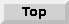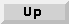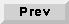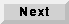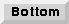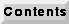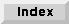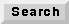7.6 An overview of DDF demos

The demos with icons shown in figure 7-7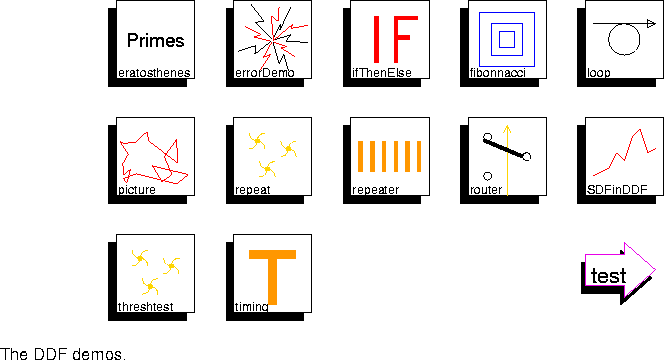illustrate dynamic dataflow principles.

eratosthenes The sieve of Eratosthenes is a recursive algorithm for computing prime numbers. This demo illustrates the implementation of recursion in the DDF domain. This is a concept demonstration only.
errorDemo An example of an inconsistent DDF system. An inconsistent DDF program is one where the long term average number of particles produced on an arc is not the same as the average long term number of particles consumed. This error is detected by bounding the buffer sizes and detecting overflow.
ifThenElse This demo illustrates the use of an SDF wormhole to implement a dynamically scheduled construct using the DDF domain. An if-then-else is such a dynamically scheduled construct. The top level schematic represents an SDF system, while the inside schematic represents a DDF system (implementing an if-then-else).
fibonnacci Generate the Fibonnacci sequence using a rather inefficient recursive algorithm that is nonetheless a good example of how to realize recursion.
loop This demo illustrates data-dependent iteration. Input integers are repeatedly multiplied by 0.5 until the product is less than 0.5. Turn on animation to see the iteration.
picture Construct a two-dimensional random walk using a hierarchy of nested wormholes. The outermost SDF domain has a wormhole called "drawline" which internally uses the DDF domain. That wormhole, in turn, has a wormhole called "display" which internally uses the SDF domain.
repeat This simple demo shows the effect of running a DDF scheduler on an SDF system. The firingsPerIteration pragma is used to control the meaning of an iteration.
repeater This is a simple illustration of the Repeater star, used in an SDF wormhole (DDF inside SDF).
router This is a simple illustration of the EndCase star.
SDFinDDF This rather trivial demo illustrates the use of a DDF wormhole whose inside domain is SDF. The top-level system (in the DDF domain) has an if-then-else overall structure, implemented of a matching pair of Case and EndCase stars. The inside system (in the SDF domain) multiplies the data value by a ramp.
threshtest This demo shows that Karp & Miller style thresholds are supported in DDF. The Thresh star is a dummy that implements a settable threshold.
timing This demo illustrates the use of the DDF domain to implement asynchronous signal processing systems. In this case, the system performs baud-rate timing recovery using an approximate minimum mean-square-error (MMSE) technique.